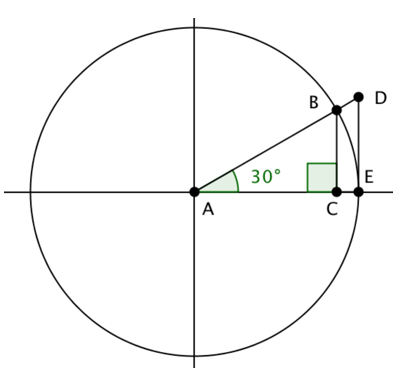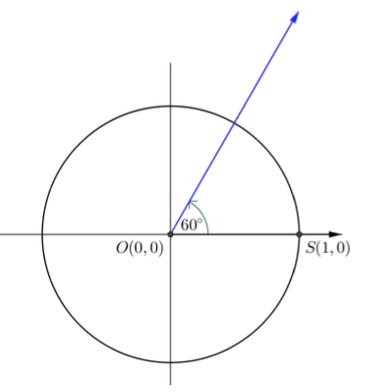# Unit Circle and Trigonometric Functions

## Objective

Describe the relationship between the unit circle and tangent.

## Common Core Standards

### Core Standards

?

• F.TF.A.2 — Explain how the unit circle in the coordinate plane enables the extension of trigonometric functions to all real numbers, interpreted as radian measures of angles traversed counterclockwise around the unit circle.

• F.TF.A.3 — Use special triangles to determine geometrically the values of sine, cosine, tangent for π/3, π/4 and π/6, and use the unit circle to express the values of sine, cosine, and tangent for π-x, π+x, and 2π-x in terms of their values for x, where x is any real number.

?

• G.SRT.C.6

## Criteria for Success

?

1. Describe tangent in terms of sine and cosine as ${\frac{\mathrm{sin{\theta}}}{\mathrm{cos{\theta}}}}$
2. Derive tangent from similar triangles in the unit circle and also as the slope of the radius at a given degree of ${\theta}$
3. Identify when the value of the tangent is undefined and describe why this is the case.

## Tips for Teachers

?

• Be prepared for students to get stuck between the ideas that there are two different definitions of tangent—one that is a ratio and one that is a length in the unit circle.
• This is the first time students are seeing a circle that is NOT a unit circle. Feel free to extend the work to non-unit circles, but be prepared to describe how the value of the tangent is scaled by the value of the radius.
• Here is a Desmos tool with the unit circle. It is helpful for demonstration purposes.
• This GeoGebra tool, The Reciprocal Trig Functions on the Unit Circle, is helpful for identifying the lengths of the trigonometric ratios and how they relate in a unit circle.

## Anchor Problems

?

### Problem 1

Given that ${\mathrm{cos\theta}=\frac{\mathrm{adjacent}}{\mathrm{hypotenuse}}}$and ${\mathrm{sin\theta}=\frac{\mathrm{opposite}}{\mathrm{hypotenuse}}}$, what is the definition of ${\mathrm{tan\theta}}$ in terms of sine and cosine?

### Problem 2

What is the slope of the line containing ${\overline{AD}}$?Show how you determine this using the trigonometric ratios for ANY angle.

### Problem 3

Below is a diagram with a line segment tangent to the circle at point $E$$\bigtriangleup ABC\approx \bigtriangleup ADE$. The radius of the circle is $1$.What is the value of $\overline{DE}$ in terms of trigonometric ratios?

## Problem Set

?

The following resources include problems and activities aligned to the objective of the lesson that can be used to create your own problem set.

• Include problems providing different representations and asking students to find the value of tangent at a specific angle.

Draw and label a figure on the circle that illustrates the relationship of the trigonometric tangent functions ${\mathrm{tan}=\frac{\mathrm{sin}(\theta^\circ)}{\mathrm{cos}(\theta^\circ)}}$ and the geometric tangent line to a circle through the point ${(1,0)}$ when ${\theta=60}$. Explain the relationship, labeling the figure as needed.